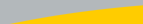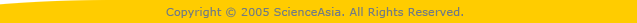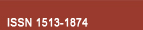| Home  | About ScienceAsia  | Publication charge  | Advertise with us  | Subscription for printed version  | Contact us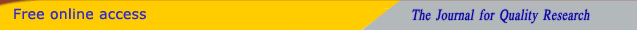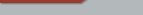Editorial Board Journal Policy Instructions for Authors Online submission Author Login Reviewer Login Volume 46 Number 3 Volume 46 Number 2 Volume 46 Number 1 Volume 46S Number 1 Volume 45 Number 6 Volume 45 Number 5 Earlier issuesVolume 45 Number 3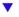Volume 45 Number 4previous article next article1

Research articles

ScienceAsia 45 (2019): 388-394 |doi: 10.2306/scienceasia1513-1874.2019.45.388

# A note on differential-difference analogue of Brück conjecture

## Minfeng Chena,*, Ning Cuib

ABSTRACT:     In this paper, we prove that for a transcendental entire function ƒ (z) of finite order, η ∈ C\\{0} is a constant such that ∆ η ƒ (z) = ƒ (z+η)− ƒ (z) ≢0, b(z) is an entire function such that σ(b) < σ(ƒ ) and λ(ƒ −b) < σ(ƒ ), if ∆η ƒ (z) and ƒ 0(z) share a(z) CM, where a(z) is an entire function satisfying σ(a) < σ(ƒ ), thenwhere a(z) and b(z) are entire functions with max{σ(a),σ(b)} < 1, H(z)(≢ 0) is an entire function with λ(H) = σ(H) < 1 and A, c,η ∈ C\\{0} are constants satisfying e = 1 + Ac. Our results are improvements and complements of those in [Bull Korean Math Soc 51 (2014) 1453-1467] and [Commun Korean Math Soc 32 (2017) 361-373].